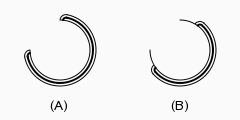# OEDrawRaceTrackSurfaceArc¶

bool OEDrawRaceTrackSurfaceArc(OEDepict::OEImageBase &image,
const OEDepict::OE2DPoint &center,
double bgnAngle, double endAngle, double radius,
const OEDepict::OEPen &pen,
double edgeAngle=10.0)


Draws an arc with the OESurfaceArcStyle_RaceTrack style.OEDrawRaceTrackSurfaceArc

image
The image on which the arc is drawn.
center
The center of the arc.
bgnAngle, endAngle
The two endpoints of the arc. Both angles are in degrees and their values have to be in a range of $$[0.0^{\circ} - 360.0^{\circ}]$$. Angles are interpreted such that $$0.0^{\circ}$$ and $$360.0^{\circ}$$ degrees are at the 12 o’clock position, $$90.0^{\circ}$$ degrees corresponds to 3 o’clock, etc.